Published

# Bike cluster

BeginnerWork in progress3 hours261## Things used in this project

### Hardware components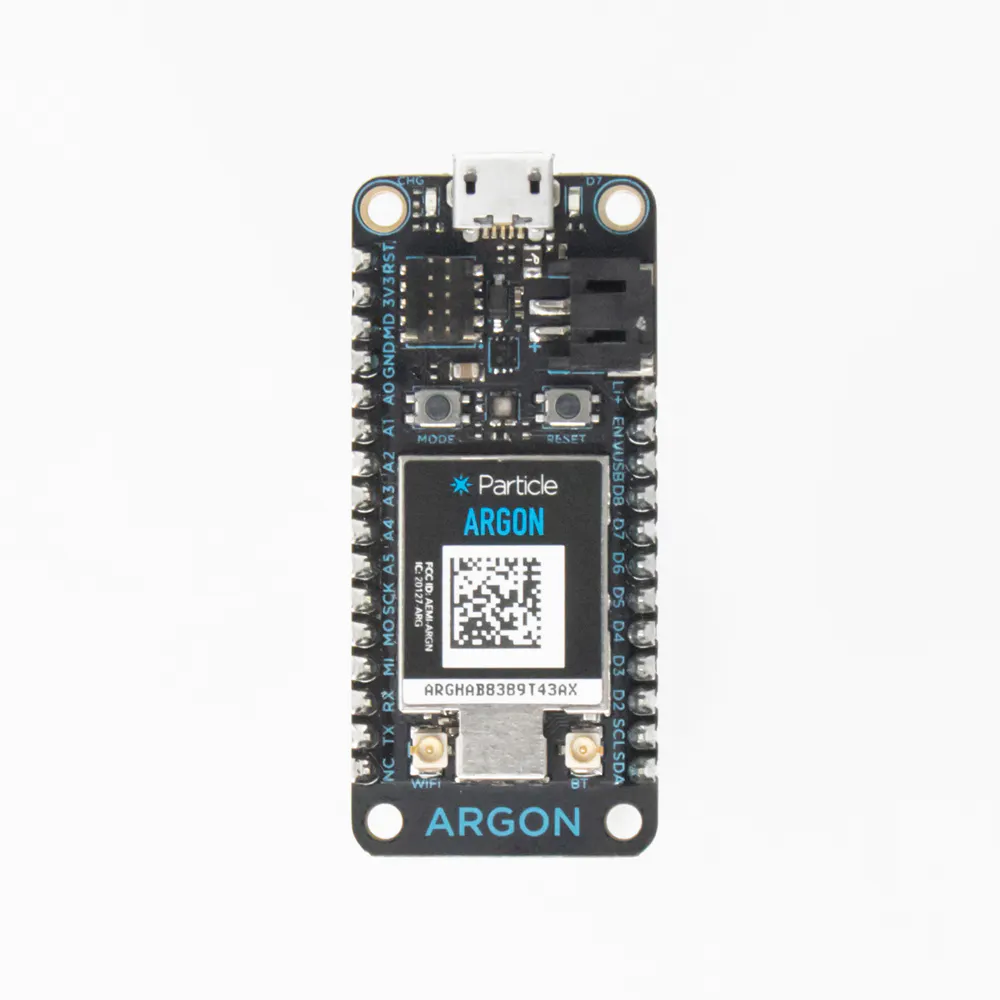Particle Argon
×2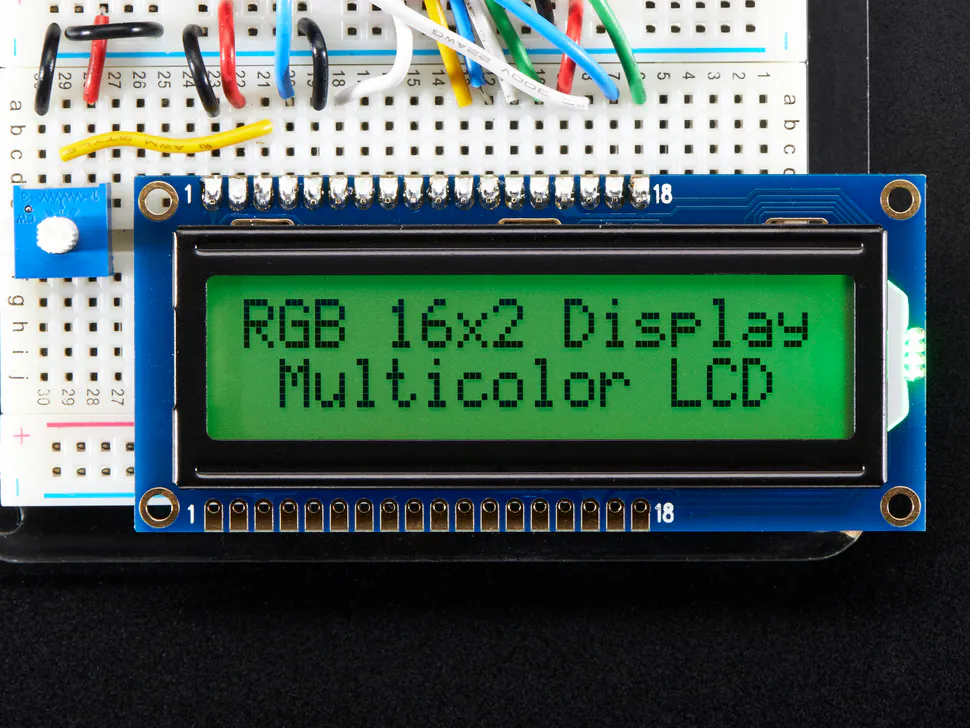Adafruit RGB Backlight LCD - 16x2
×1Jumper wires (generic)
×2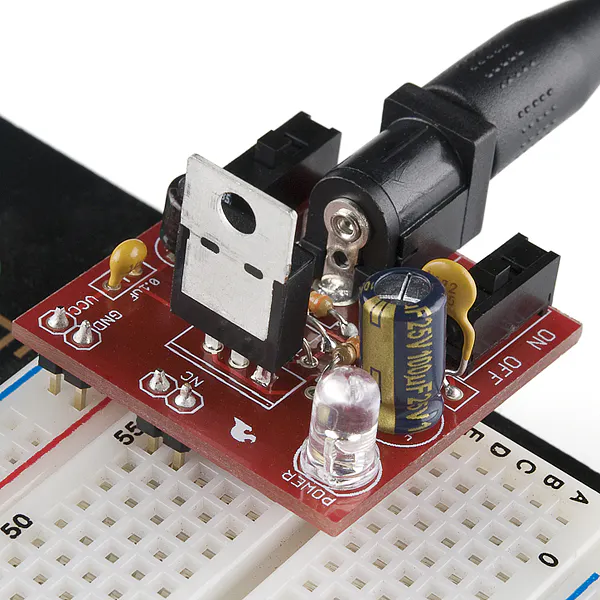×1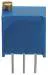Multi-Turn Precision Potentiometer- 10k ohms (25 Turn)
×1×1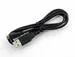USB-A to Micro-USB Cable
×1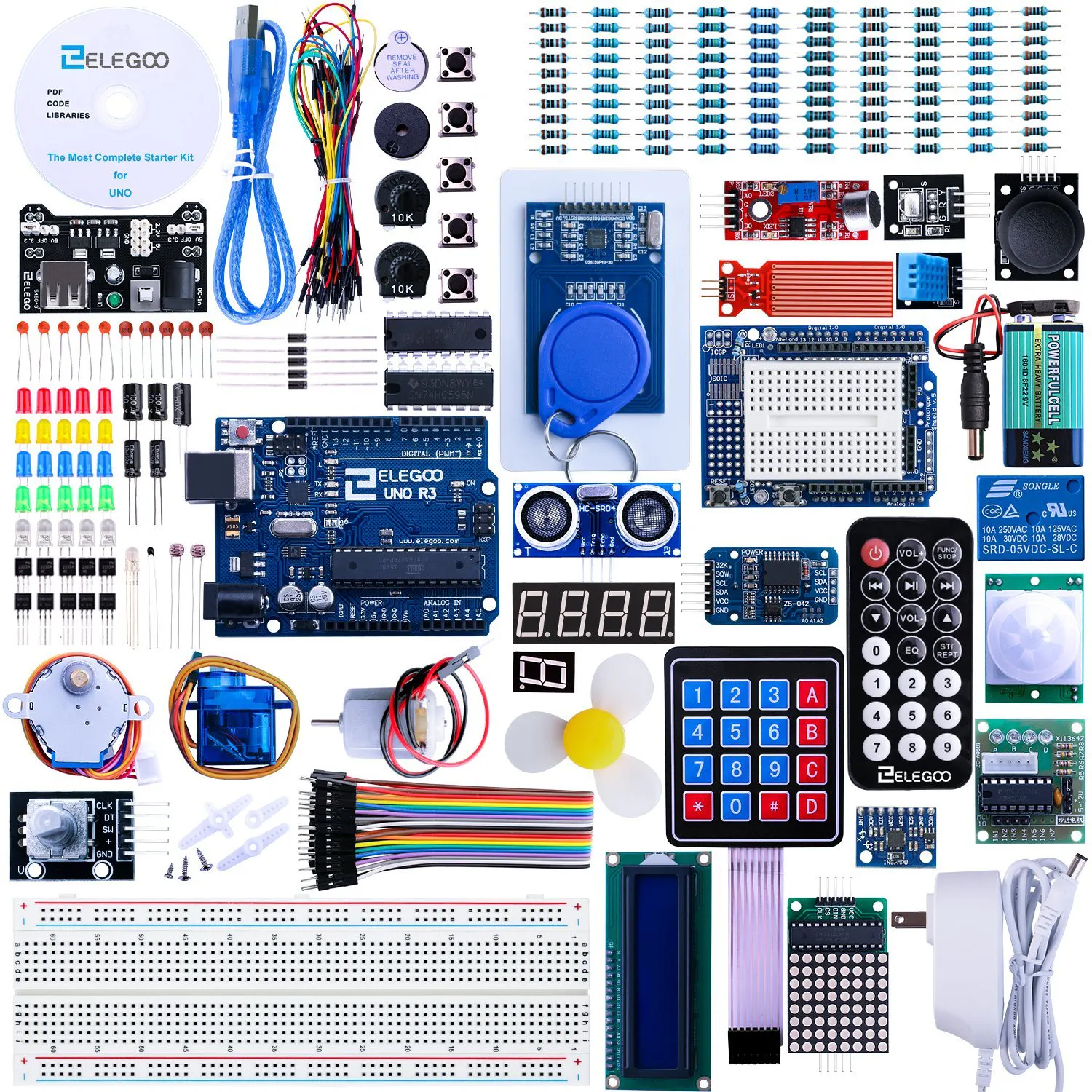ELEGOO UNO R3 Project Complete Starter Kit
×1
 Bike Magnet
×1

### Software apps and online servicesParticle Build Web IDEThingSpeak API

## Schematics

### Magnetic Spring Circuit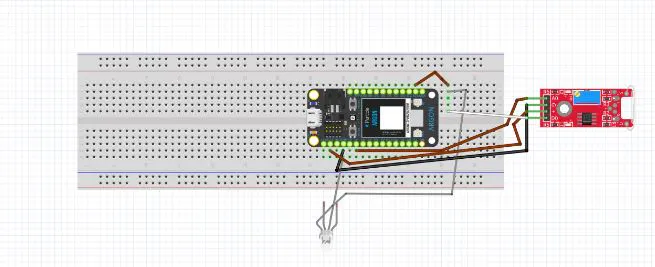### LCD + Temperature Sensor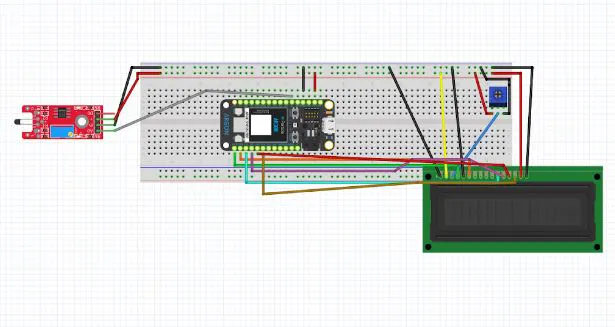## Code

### Magnetic spring

C/C++
```// This #include statement was automatically added by the Particle IDE.
#include <ThingSpeak.h>

int ANALOG_MAG_PIN = A1; //The analog pin for the analog output of the sensor
int DIGITAL_MAG_PIN = D1; //The digital pin for the digital output of the sensor
int Speed; //The speed variable
int timer; //Time Variable
int totaltime; // The total time declared for magnet to travel
int circumference; //Circumference Variable
int mag_D; //Variable to store the digital reading
int mag_A; //Variable to store the analog reading
const char * writeKey = "C8KM44MU1C1QYS21"; //Writekey allows for data to be plotted
unsigned long channel = 1915324;
TCPClient client;

void Start()
{
timer++;                        // Begin the timer and start counting
delayMicroseconds(9600);        // Delay to start after a 0.0096 seconds
}

void setup()
{
Serial.begin(9600); //Start the serial connection to the computer

attachInterrupt(D1, Start, RISING); // Begins the counting when the reading from D1 is Low

pinMode(ANALOG_MAG_PIN, INPUT); //Make the pin you used an input on the Argon
pinMode(DIGITAL_MAG_PIN, INPUT); //Make the pin you used an input on the Argon
timer = 0;                                                 // Sets timer to 0 seconds
Speed = 0;                                                 // sets the speed to 0 seconds
totaltime = 0;                                             // sets the total time to start off at 0
circumference = 6.28 * radius;                             // calculated the circumference
ThingSpeak.begin(client);

}

//This code will run infinitely
void loop()
{
if (millis() - totaltime >= 1000) {  // Gets varibales to go into the loop everytime the millis() - the total time elapsed is less than 1ms
detachInterrupt(D1);
Speed = ((8.181818182*circumference)/(millis() - totaltime)*timer); // Calculates the speed of the bike. The 8.18 number is converting the time in seconds into hours and inches into feet.

timer = 0;                                                          // Makes the timer go back to zero
totaltime = millis();                                               // Makes the total time the new time
attachInterrupt(D1, Start, RISING);             // Make the Start loop attach back

delay(1000);
if(mag_D == HIGH)
{

Particle.publish("Speed", String(Speed),ALL_DEVICES); //Publishes the velocity of the bike in MPH
ThingSpeak.setField(1, Speed);
ThingSpeak.writeFields(channel, writeKey);

}
else
{
int speed=0;
Particle.publish("Speed", String(Speed),ALL_DEVICES); //Publishes the velocity of the bike in MPH
ThingSpeak.setField(1, Speed);
ThingSpeak.writeFields(channel, writeKey);
}
}

}
```

### Lcd + Temp sensor

C/C++
```// This #include statement was automatically added by the Particle IDE.
#include <ThingSpeak.h>

// This #include statement was automatically added by the Particle IDE.
#include "application.h"
#include <LiquidCrystal.h>
const char velocity=0;
double temp = 0;
int tits = 0;
LiquidCrystal lcd (D0,D1,D2,D3,D4,D5);
double analogvalue=0;
const String writeKey = "C8KM44MU1C1QYS21"; //Writekey allows for data to be plotted
unsigned long channel = 1915324;
TCPClient client;
int temperature;
int i=0;

void setup()
{
Particle.subscribe("Speed", myHandler, "e00fce689d7bea81502f393c");
lcd.clear();
lcd.begin(16, 2);
lcd.setCursor(0,0);
lcd.print("Temp: ");

lcd.setCursor(0,1);
lcd.print("speed: ");

pinMode(D7, OUTPUT);
delay(500);
digitalWrite(D7,LOW);

Particle.variable("analogvalue", analogvalue);
Particle.variable("temp",temp);
Particle.variable("time", tits);

pinMode(A1, INPUT );
tits = 0;

ThingSpeak.begin(client);
}

void myHandler(const char *event, const char *data){

lcd.setCursor(6,1);
lcd.print(data);
lcd.setCursor(9,1);
lcd.print(" mph ");
delay(1000);

}

void loop() {

delay(2000);

temp = (analogvalue*-.08099688473520249+151.99688473520249);

Serial.begin(9600);
temperature =temp;
lcd.setCursor(5,0);
lcd.print(temp);
lcd.setCursor(9,0);
lcd.print(" F ");

//publish the data to partner argon

Particle.publish("Temperature", String(temp));
ThingSpeak.setField(2,temperature);
ThingSpeak.writeFields(channel, writeKey);

delay(1000);

temp = 0;
tits = tits + 1000;
if(tits > 5000)
{
tits = 0;
///////////////////////////////Start Of Velocity Code////////////////////////////////////

}

}
```